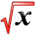# More MathPracticeThis article is part of the MathHelp Tutoring Wiki

## Rates

Q Liquid is being poured into a parabolic bowl at a constant rate of $60\pi cm^{3}/s$. The volume of the bowl is given by $V=(1/2)\pi x^{4}$, where the equation of the parabola is $y=x^{2}$, where y is the height of liquid in the bowl. Find the rate of increase of the height of the liquid in the bowl when the height is 10 centimetres.

A We can write Volume $V=(1/2)\pi x^{4}=(1/2)\pi y^{2}$${\frac {dV}{dt}}=\pi y{\frac {dy}{dt}}$${\frac {dV}{dt}}$is $60\pi$.

So, when y=10, we can evaluate ${\frac {dy}{dt}}$which is the rate of change, which evaluates to be 6cm/s.